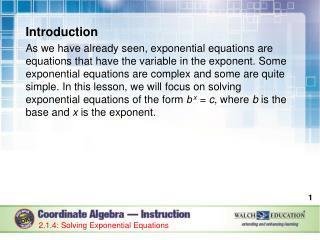DownloadDownload PresentationIntroduction

# Introduction

Télécharger la présentation## Introduction

- - - - - - - - - - - - - - - - - - - - - - - - - - - E N D - - - - - - - - - - - - - - - - - - - - - - - - - - -
##### Presentation Transcript

1. Introduction As we have already seen, exponential equations are equations that have the variable in the exponent. Some exponential equations are complex and some are quite simple. In this lesson, we will focus on solving exponential equations of the form b x = c, where b is the base and x is the exponent. 2.1.4: Solving Exponential Equations

2. Key Concepts It may help to look at the laws of exponents. These laws, sometimes referred to as properties, are the rules that must be followed when working with exponents. The following table summarizes these laws. 2.1.4: Solving Exponential Equations

3. Key Concepts, continued Laws of Exponents 2.1.4: Solving Exponential Equations

4. Key Concepts, continued • Keep these laws in mind when solving exponential equations. • There are two forms of exponential equations. One form is used when each side of the equation can be written using the same base, such as ab = ac. In this case, b and c must be equal as long as a > 0 and a ≠ 1. • The second form of exponential equations is used when it isn’t possible to write each side of the equation using the same base. How to solve this type of exponential equation will be covered in a later lesson. 2.1.4: Solving Exponential Equations

5. Key Concepts, continued Follow a few basic guidelines to solve an exponential equation where the bases of both sides of the equation can be written so that they are equal. 2.1.4: Solving Exponential Equations

6. Common Errors/Misconceptions attempting to solve an exponential equation as if it is a linear equation not finding a common base prior to attempting to solve the equation misidentifying the common base 2.1.4: Solving Exponential Equations

7. Guided Practice Example 1 Solve 4x= 1024. 2.1.4: Solving Exponential Equations

8. Guided Practice: Example 1, continued Rewrite the base as powers of a common base. You may not recognize right away if it is possible to write 1,024 as an exponential expression with a base of 4. Begin by finding values of powers of 4 to see if it is possible. 41 = 4 44 = 256 42 = 16 45= 1024 43= 64 We now know that it is possible to write 1,024 as a power of 4. 2.1.4: Solving Exponential Equations

9. Guided Practice: Example 1, continued Rewrite the equation so that both sides have a base of 4. 4x= 45 2.1.4: Solving Exponential Equations

10. Guided Practice: Example 1, continued Now solve for x by setting the exponents equal to each other. x = 5 The solution to the equation 4x= 1024 is x = 5. 2.1.4: Solving Exponential Equations

11. Guided Practice: Example 1, continued Check your answer. Substitute 5 for the variable x in the original equation. 4x= 1024 45 = 1024 1024 = 1024 This is a true statement. ✔ 2.1.4: Solving Exponential Equations

12. Guided Practice: Example 1, continued 2.1.4: Solving Exponential Equations

13. Guided Practice Example 4 Solve the equation 117 = 5x – 8. 2.1.4: Solving Exponential Equations

14. Guided Practice: Example 4, continued Begin by eliminating the subtraction of 8 from the right side of the equal sign. Do so by adding 8 to both sides of the equation. 2.1.4: Solving Exponential Equations

15. Guided Practice: Example 4, continued Rewrite the base as powers of a common base. 125 can be written as 5 to the power of 3. 2.1.4: Solving Exponential Equations

16. Guided Practice: Example 4, continued Rewrite the equation so both sides have a base of 5. 125 = 5x 53 = 5x 2.1.4: Solving Exponential Equations

17. Guided Practice: Example 4, continued Now solve for x by setting the exponents equal to each other. x = 3 The solution to the equation 117 = 5x – 8 is x = 3. 2.1.4: Solving Exponential Equations

18. Guided Practice: Example 4, continued Check your answer. Substitute 3 for the variable x in the original equation. 117 = 5x – 8 117 = 53 – 8 117 = 125 – 8 117 = 117   This is a truestatement. ✔ 2.1.4: Solving Exponential Equations

19. Guided Practice: Example 4, continued 2.1.4: Solving Exponential Equations